# RD Sharma Class 8 Solutions Chapter 2 Powers Ex 2.2

## RD Sharma Class 8 Solutions Chapter 2 Powers Ex 2.2

These Solutions are part of RD Sharma Class 8 Solutions. Here we have given RD Sharma Class 8 Solutions Chapter 2 Powers Ex 2.2

Other Exercises

Question 1.
Write each of the following in exponential form :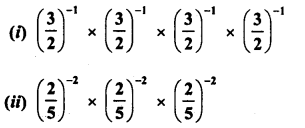Solution: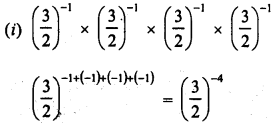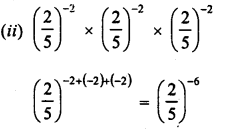Question 2.
Evaluate :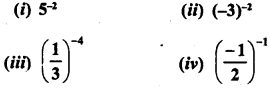Solution: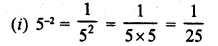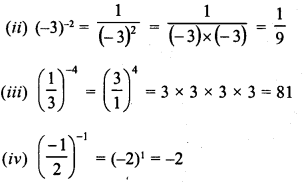Question 3.
Express each of the following as a rational number in the form $$\frac { p }{ q } :$$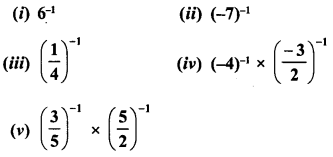Solution: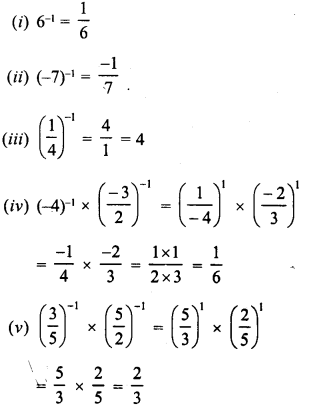Question 4.
Simplify :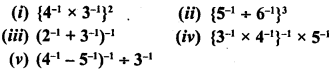Solution: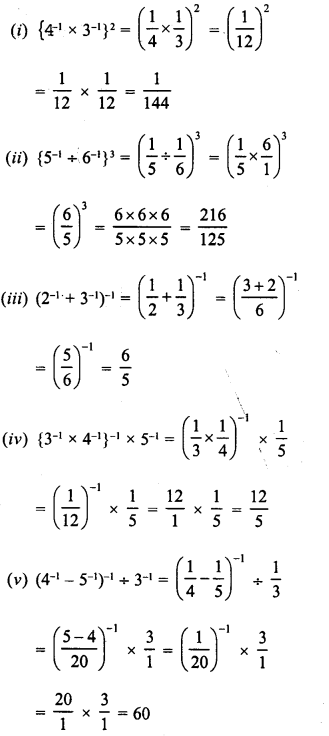Question 5.
Express each of the following rational numbers with a negative exponent :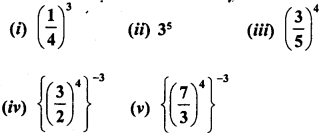Solution: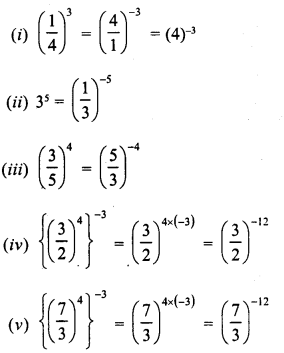Question 6.
Express each of the following rational numbers with a positive exponent :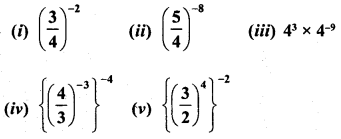Solution: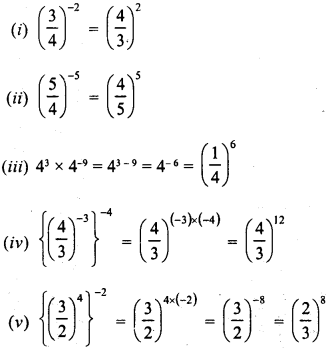Question 7.
Simplify :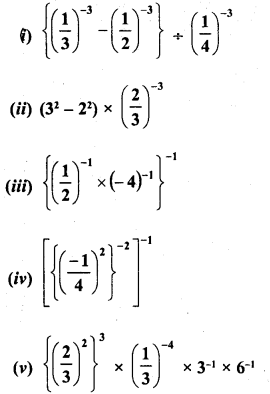Solution: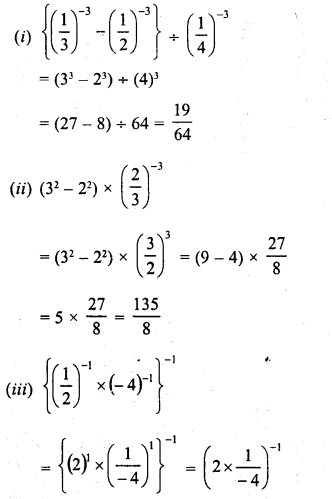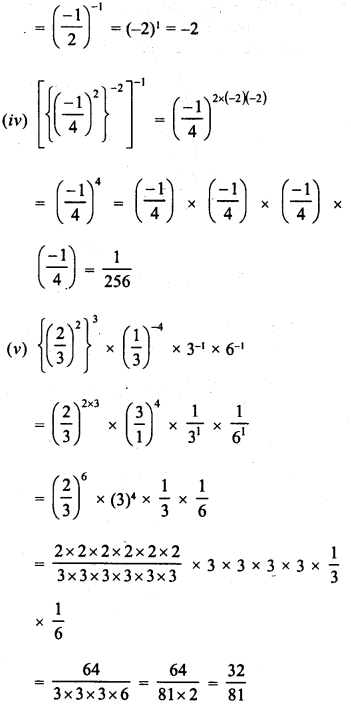Question 8.
By what number should 5-1 be multiplied so that the product may be equal to (-7)-1 ?
Solution: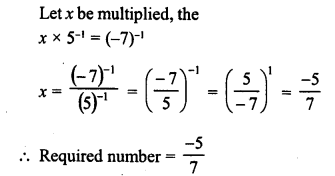Question 9.
By what number should $${ \left( \frac { 1 }{ 2 } \right) }^{ -1 }$$ be multiplied so that the product may be equal to $${ \left( \frac { -4 }{ 7 } \right) }^{ -1 }$$ ?
Solution: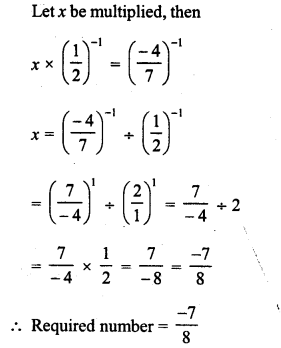Question 10.
By what number should (-15)-1 be divided so that the quotient may be equal to (-5)-1 ?
Solution: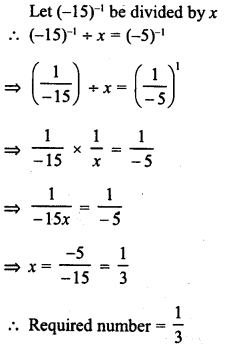Question 11.
By what number should $${ \left( \frac { 5 }{ 3 } \right) }^{ -2 }$$ be multiplied so that the product may be $${ \left( \frac { 7 }{ 3 } \right) }^{ -1 }$$ ?
Solution: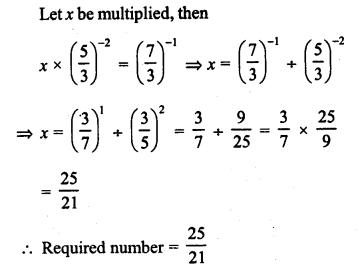Question 12.
Find x, if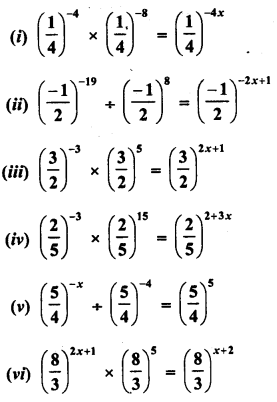Solution: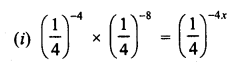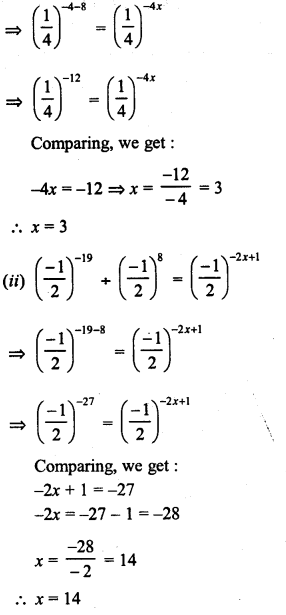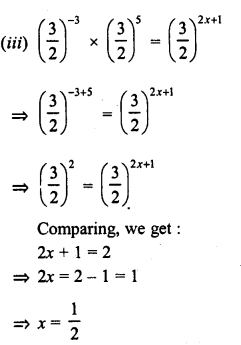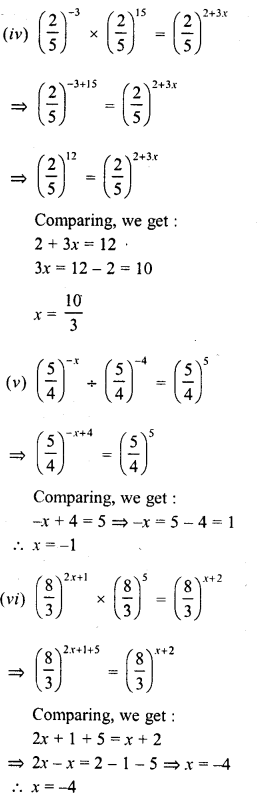Question 13.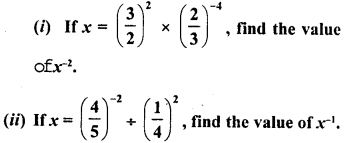Solution: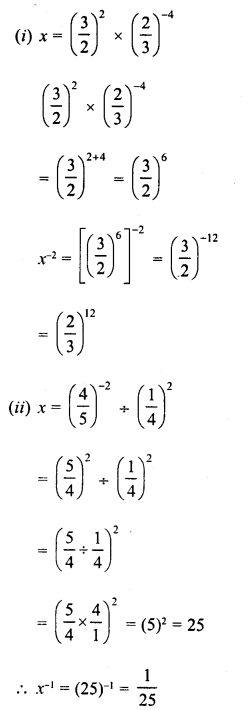Question 14.
Find the value of x for which 52x + 5-3 = 55.
Solution: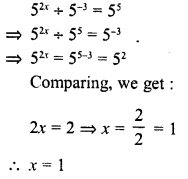Hope given RD Sharma Class 8 Solutions Chapter 2 Powers Ex 2.2 are helpful to complete your math homework.

If you have any doubts, please comment below. Learn Insta try to provide online math tutoring for you.